### Home > CC2MN > Chapter 1 > Lesson 1.2.1 > Problem1-59

1-59.

Add or subtract.  Remember to line up the decimal points.1. $53.199−27.61$

Line the problem up vertically. Numbers arranged in 2 rows of 7 columns as follows: Top row: 5, 3, decimal point, 1, 9, 9. Bottom row: 2, 7, decimal point, 6, 1, blank.

Add a zero and subtract. Digit 0 added under last 9. Answer has 9 in far right column.

Continue subtracting going from right to left. Answer has 8, left of 9.

Borrow 1 from the 3. Then subtract 6 from 11. Changes: top row, 3 to 2, 1 to 11, answer add 5 left of 8.

Borrow again. Changes: top row, 5 to 4, 2 to 12.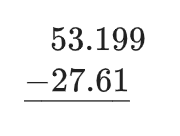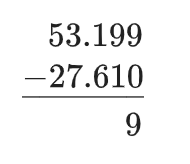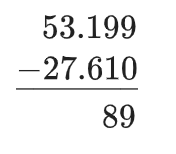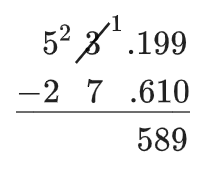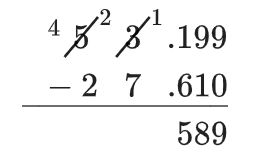2. $155.96+56.232$

The 2 numbers are stacked in vertical columns as follows: 1 and blank, 5 and 5, 5 and 6, decimal point and decimal point, 9 and 2, 6 and 3, blank and 2.

Add from right to left, carrying when necessary. Now finish the problem. Changes: top row: 1 above 5 in 3rd column and 0 in last column, answer: first 3 columns are blank, then decimal point, 1, 9, and 2.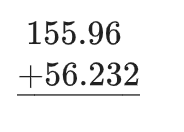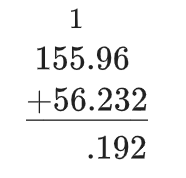3. $83.617−36.518$

See part (a).